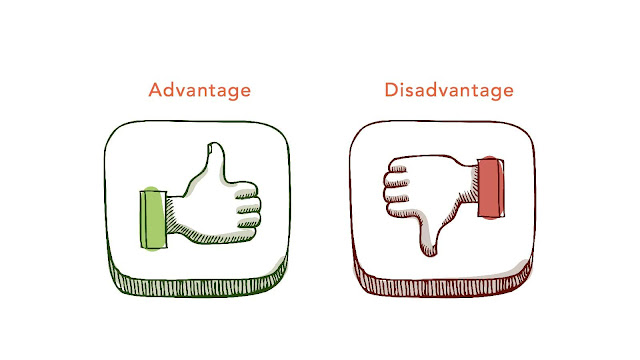# HSSlive: Plus One & Plus Two Notes & Solutions for Kerala State Board

## Monday, March 13, 2023Advantages And Disadvantages Of 2D Rectangular Array

## What is 2D Rectangular Array?

A 2D rectangular array, also known as a 2D matrix or 2D array, is a data structure that stores values in a two-dimensional grid or table. It is composed of rows and columns, and each element in the array is identified by its row and column index. The values in a 2D rectangular array are typically homogeneous, meaning that they are all of the same data type.

## Advantages of 2D Rectangular Array

Some advantages of using a 2D rectangular array include:

1. Efficient storage: A 2D rectangular array can store large amounts of data in a compact and organized manner.

2. Easy indexing: Elements in a 2D rectangular array can be accessed using their row and column indices, making it easy to access specific data points.

3. Flexibility: 2D rectangular arrays can be resized dynamically during program execution to accommodate changes in data size.

4. Matrix operations: 2D rectangular arrays can be used to perform matrix operations such as addition, multiplication, and transposition, which are important in fields such as data science, machine learning, and engineering.

5. Easy visualization: 2D rectangular arrays can be easily visualized as tables, making it easier for developers to understand the data and identify patterns.

Overall, 2D rectangular arrays are a powerful data structure that provide a flexible and efficient way to store and manipulate large amounts of data.

## Disadvantages of 2D Rectangular Array

One of the main disadvantages of a 2D rectangular array is that it is inflexible. Once created, the size of the array cannot be changed easily. If the number of elements needed in the array changes, the programmer may need to create a new array and copy over the data from the old array.

Another disadvantage is that the rectangular shape may not always be the best choice for representing certain types of data. For example, if the data is better represented as a tree or graph structure, a rectangular array may not be the most efficient or intuitive choice.

Additionally, accessing individual elements in a 2D rectangular array can be slower compared to accessing elements in a one-dimensional array because the position of each element in the array must be calculated based on both its row and column index.

Share: